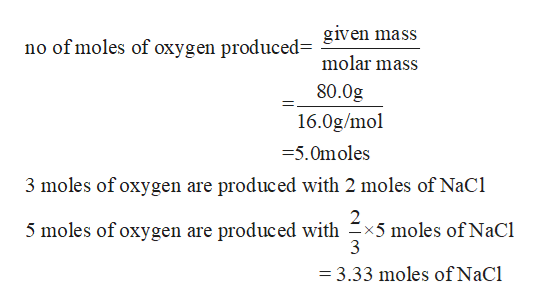# Given the following equation: 2 NaClO3 ➞ 2 NaCl + 3 O2. How many grams of NaCl are produced when 80.0 grams of O2 are produced? (Molecular mass of oxygen: 16 g/mol) (Molar mass of sodium chloride: 58.45 g/mol)

Question
85 views

Given the following equation: 2 NaClO3 ➞ 2 NaCl + 3 O2. How many grams of NaCl are produced when 80.0 grams of O2 are produced? (Molecular mass of oxygen: 16 g/mol) (Molar mass of sodium chloride: 58.45 g/mol)

check_circle

Step 1

Given

The mass of oxygen produced =80.0g

The equation according to which reaction takes place

Step 2

The moles of NaCl producedhelp_outlineImage Transcriptionclosegiven mass no of moles of oxygen produced= molar mass 80.0g 16.0g/mol =5.0moles 3 moles of oxygen are produced with 2 moles of NaCi 5 moles of oxygen are produced with x5 moles of NaCl 3 3.33 moles of NaCl fullscreen
Step 3

Mass of NaCl p...

### Want to see the full answer?

See Solution

#### Want to see this answer and more?

Solutions are written by subject experts who are available 24/7. Questions are typically answered within 1 hour.*

See Solution
*Response times may vary by subject and question.
Tagged in

### Quantitative analysis